### Home > CALC > Chapter 1 > Lesson 1.2.3 > Problem1-60

1-60.
1. When the flag below is rotated it has a volume of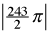un3. Homework Help ✎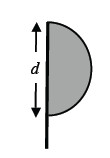1. Describe the resultant three-dimensional figure.

2. What is the value of d?

3. If the diagram was rotated 90° and the flag was then rotated about a horizontal pole, would the volume change?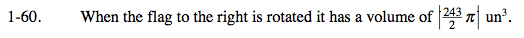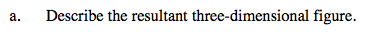Use the eTool below to visualize the rotating flag.
Click the link at right for the full version of the eTool: Calc 1-60 HW eTool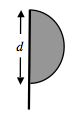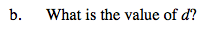$\frac{243}{2}\pi =\frac{4}{3}\pi r^{3}$

$r=\frac{1}{2}d$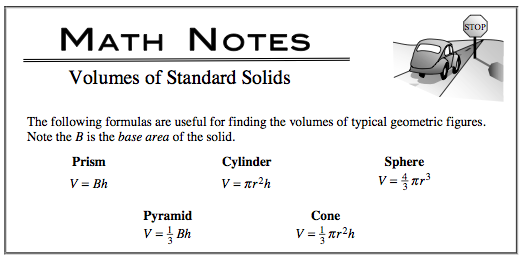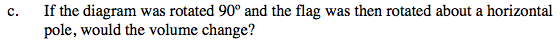Build a model by taping a paper flag to a pencil. Try holding the pencil in different positions. Does the size or shape of the resultant solid change when the pencil is held horizontally vs. vertically?